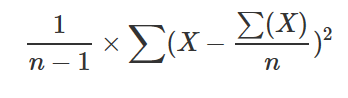# variance() (aggregation function)

Calculates the variance of Expr across the group, considering the group as a sample.

• Used formula:• Can be used only in context of aggregation inside summarize

## Syntax

`variance` `(`Expr`)`

## Arguments

• Expr: Expression that will be used for aggregation calculation.

## Returns

The variance value of Expr across the group.

## Examples

``````range x from 1 to 5 step 1
| summarize make_list(x), variance(x)
``````
list_x variance_x
[ 1, 2, 3, 4, 5] 2.5